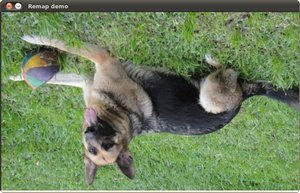OpenCV  4.1.1 Open Source Computer Vision
Remapping

Prev Tutorial: Hough Circle Transform

Next Tutorial: Affine Transformations

## Goal

In this tutorial you will learn how to:

a. Use the OpenCV function cv::remap to implement simple remapping routines.

## Theory

### What is remapping?

• It is the process of taking pixels from one place in the image and locating them in another position in a new image.
• To accomplish the mapping process, it might be necessary to do some interpolation for non-integer pixel locations, since there will not always be a one-to-one-pixel correspondence between source and destination images.
• We can express the remap for every pixel location $$(x,y)$$ as:

$g(x,y) = f ( h(x,y) )$

where $$g()$$ is the remapped image, $$f()$$ the source image and $$h(x,y)$$ is the mapping function that operates on $$(x,y)$$.

• Let's think in a quick example. Imagine that we have an image $$I$$ and, say, we want to do a remap such that:

$h(x,y) = (I.cols - x, y )$

What would happen? It is easily seen that the image would flip in the $$x$$ direction. For instance, consider the input image: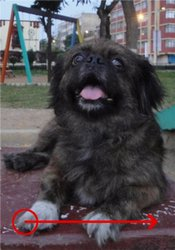observe how the red circle changes positions with respect to x (considering $$x$$ the horizontal direction):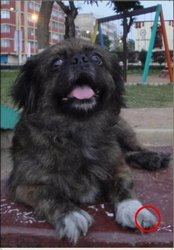• In OpenCV, the function cv::remap offers a simple remapping implementation.

## Code

• What does this program do?
• Each second, apply 1 of 4 different remapping processes to the image and display them indefinitely in a window.
• Wait for the user to exit the program

## Explanation

• Create the destination image and the two mapping matrices (for x and y )
• Create a window to display results
• Establish a loop. Each 1000 ms we update our mapping matrices (mat_x and mat_y) and apply them to our source image:
• The function that applies the remapping is cv::remap . We give the following arguments:

• src: Source image
• dst: Destination image of same size as src
• map_x: The mapping function in the x direction. It is equivalent to the first component of $$h(i,j)$$
• map_y: Same as above, but in y direction. Note that map_y and map_x are both of the same size as src
• INTER_LINEAR: The type of interpolation to use for non-integer pixels. This is by default.
• BORDER_CONSTANT: Default

How do we update our mapping matrices mat_x and mat_y? Go on reading:

• Updating the mapping matrices: We are going to perform 4 different mappings:
1. Reduce the picture to half its size and will display it in the middle:

$h(i,j) = ( 2 \times i - src.cols/2 + 0.5, 2 \times j - src.rows/2 + 0.5)$

for all pairs $$(i,j)$$ such that: $$\dfrac{src.cols}{4}<i<\dfrac{3 \cdot src.cols}{4}$$ and $$\dfrac{src.rows}{4}<j<\dfrac{3 \cdot src.rows}{4}$$
2. Turn the image upside down: $$h( i, j ) = (i, src.rows - j)$$
3. Reflect the image from left to right: $$h(i,j) = ( src.cols - i, j )$$
4. Combination of b and c: $$h(i,j) = ( src.cols - i, src.rows - j )$$

This is expressed in the following snippet. Here, map_x represents the first coordinate of h(i,j) and map_y the second coordinate.

## Result

1. After compiling the code above, you can execute it giving as argument an image path. For instance, by using the following image: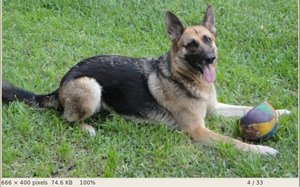2. This is the result of reducing it to half the size and centering it: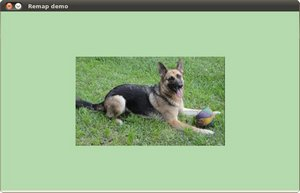3. Turning it upside down: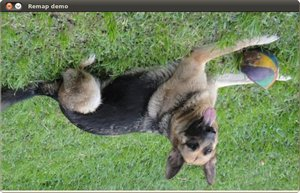4. Reflecting it in the x direction: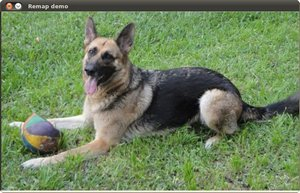5. Reflecting it in both directions: